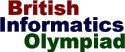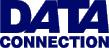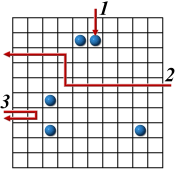The British Informatics Olympiad is the computing competition for schools and colleges. We are proud to present BIO'99.The 1999 British Informatics Olympiad - Round One

Time allowed: 3 hours

Instructions

You should write a program for part (a) of each question, and produce written answers to the remaining parts. Programs may be used to help produce the answers to these written questions. You may use a calculator and the on-line help that your programming language provides, and you should have a pen, some blank paper, and a blank floppy disk on which to save your programs. You must not use any other material such as disks, files on a computer network, books or other written information.

Mark the first page used for your written answers with your name, age in years and school/college. Number all pages in order if you use more than one sheet. All of your computer programs should display your name and school/college when they are run, and the floppy disk you use to submit the programs should also show your name and school/college.

For your programs to be marked, the source code must be saved, along with executables if your language includes a compiler. This includes programs used to help answer written questions. You must clearly indicate the name given to each program on your answer sheet(s).

Sample runs are given for parts 1(a), 2(a) and 3(a). ```Bold text``` indicates output from the program, and ```normal text``` shows data that has been entered. The output format of your programs should follow the 'sample run' examples. Your programs should take less than two minutes of processing time for each test.

Attempt as many questions as you can. Marks allocated to each part are shown in square brackets next to the questions. Partial solutions (such as programs that only satisfy some of the specified requirements, or partly completed written answers) may get partial marks. You may answer the questions in any order.Question 1 Digital rivers A digital river is a sequence of numbers where the number following n is n plus the sum of its digits. For example, 12345 is followed by 12360, since 1+2+3+4+5 = 15. If the first number of a digital river is k we will call it river k.For example, river 480 is the sequence beginning {480, 492, 507, 519, ...} and river 483 is the sequence beginning {483, 498, 519, ...}. Normal streams and rivers can meet, and the same is true for digital rivers. This happens when two digital rivers share some of the same values. For example: river 480 meets river 483 at 519, meets river 507 at 507, and never meets river 481. 1 (a) [20 marks] Every digital river will eventually meet river 1, river 3 or river 9. Write a program which inputs a single integer n (1<=n<=16384), and outputs the value where river n firstmeets one of these three rivers. Sample run River: 86 First meets river 1 at 101
 1 (b) [5 marks] What is the lowest number that can be found in exactly 100 digital rivers? [For example, 8 is the lowest number in 4 rivers: rivers 1, 2, 4, and 8.]Question 2 Black Box (Atom)Scientists are studying an enigmatic Black Box by firing rays into it and observing the results. The rays are influenced by atoms within the box. Your task for this question is to model the rays' behaviour.The box is a 10 by 10 grid. Each square is either empty or contains a single atom. The 36 squares on the boundary of the box, i.e. the squares adjacent to an outside edge, never contain atoms. Rays are fired into the box from a point on the grid's edge, and can travel horizontally and vertically. Rays continue travelling in straight lines, unless affected by atoms as follows: If the ray hits an atom it is absorbed (example ray 1). If the ray is about to pass though a square next to an atom, it is deflected by 90 degrees away from the atom (example ray 2). A ray that would pass between two atoms one square apart is reflected (example ray 3). These rules are given in order of precedence. Note that sample ray 1 is absorbed rather than deflected.
 2 (a) [25 marks] Write an interactive program to show how the rays are affected by a black box containing five atoms.The entry and exit points for rays will be given by a single letter followed by an integer. The letter, 'T', 'B', 'L' or 'R', indicates the side of the grid: top, bottom, left or right. The number indicates the position on the given side; for the top and bottom sides, 1 is on the left; for the left and right sides, 1 is at the bottom. Your program should first read in 5 lines each containing two numbers; the x co-ordinate then the y co-ordinate for an atom. The bottom left corner is (1,1). You should then output the black box - using '.' for an empty square and 'A' for one containing an atom. Until your program terminates you should repeatedly wait for input, and then: If you receive 'T', 'B', 'L' or 'R' followed by an integer between 1 and 10, you should treat it as the entry point for a ray. You should display the black box, using a '+' to indicate any square the ray enters which was empty, and a '*' for any it enters that contained an atom. If the ray was absorbed or reflected you should output the corresponding word; otherwise you should output the exit point. If you receive the input "X 0" you should terminate. Ignore any other input. Sample run 3 3 9 3 3 5 5 9 6 9   .......... ....AA.... .......... .......... .......... ..A....... .......... ..A.....A. .......... ..........   T 6 .....+.... ....A*.... .......... .......... .......... ..A....... .......... ..A.....A. .......... .......... Absorbed   R 6 .......... ....AA.... ++++...... ...+...... ...+++++++ ..A....... .......... ..A.....A. .......... .......... Exits at L 8   L 4 .......... ....AA.... .......... .......... .......... ..A....... ++........ ..A.....A. .......... .......... Reflected   T 8 .......+.. ....AA.+.. .......+.. .......+.. .......+.. ..A....+.. ...+++++.. ..A.....A. .......... .......... Reflected   X 0
 2 (b) [3 marks] What is the smallest number of atoms that can be placed in an empty black box, so that all 40 possible rays will be affected? Draw a suitable arrangement to illustrate your answer. 2 (c) [4 marks] A ray is fired into a box at T 5 and emerges at B 5. If there are four atoms inside the box, how many different paths might that ray have taken? 2 (d) [8 marks] A ray might pass through a square once (e.g. squares on the path of sample ray 2) or twice (e.g. squares on the path of sample ray 3). How about exactly 3 times? How about exactly 4 times? Justify your answers.Question 3 Playing games Romulus is playing a game which is divided into rounds. At the end of each round points are awarded depending on the result, and at the end of the game these points are summed for the final score.Given a final score, Romulus is interested in finding the minimum number of rounds that might have taken place. For example: 3 or 5 points are awarded at the end of each round, and Romulus finished with a score of 15. The minimum number of rounds is 3 (each scoring 5). Note that some scores, such as 4, are impossible to obtain in this case.
 3 (a) [26 marks] Write a program that inputs a list of possible points that can be awarded in a round, followed by a list of final scores. For each final score you should output the minimum number of rounds that might have taken place, along with the corresponding points scored.The first line of input will consist of a single integer n (1<=n<=10) denoting the number of possible points that can be scored in a round. The second line will consist of n integers (between 1 and 500) giving the possible points. Note that the numbers in the second line will not necessarily be sorted. The third line of input will be a single integer m (1<=m<=10) indicating the number of final scores to be considered. The fourth line will give the m final scores to be considered (between 1 and 1,000). Your output should consist of m lines, one for each of the final scores. Each line should contain the minimum number of rounds, followed by a corresponding example set of points. You should follow the format given in the sample run; note that the example set does not need to be sorted. If it is not possible to produce a given score you should just output Impossible on that line. Sample run 6 50 10 2 5 1 20 3 10 49 101 1 1x10 5 1x5 2x20 2x2 3 2x50 1x1
 3 (b) [4 marks] Romulus is playing a game where it is possible to score 1, 3, 9, 27 or 81, and after he is finished Remus also plays the same game. When they compare their final scores Romulus has scored 80 more than Remus. What is the minimum number of rounds they may have played between them? How about if Romulus has 50 more points than Remus? [Give an example in each case.] 3 (c) [5 marks] Remus is playing a game where it is possible to score 1, 4, 5, 17, 28, 43 or 100 each round. At the end of the game the final score is 100. Furthermore, the scores for each round never got worse, e.g. if 17 was scored in one round then the score for every future round was at least 17. How many different ways might this have happened?

Total marks: 100.
End of BIO'99 Round One paper

The British Informatics Olympiad